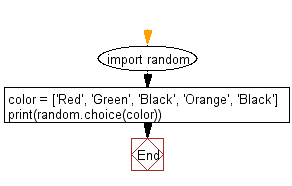﻿ Python Math: Randomly select an item from a list - w3resource# Python Math: Randomly select an item from a list

## Python Math: Exercise-55 with Solution

Write a Python program to randomly select an item from a list.

Sample Solution:-

Python Code:

``````import random
color = ['Red', 'Green', 'Black', 'Orange', 'Black']
print(random.choice(color))
```
```

Sample Output:

```Black
```

Pictorial Presentation:Flowchart:## Visualize Python code execution:

The following tool visualize what the computer is doing step-by-step as it executes the said program:

Python Code Editor:

Have another way to solve this solution? Contribute your code (and comments) through Disqus.

What is the difficulty level of this exercise?

Test your Python skills with w3resource's quiz

﻿

## Python: Tips of the Day

Python: Unpack a Tuple

```>>> items = (0, 'b', 'one', 10,  11, 'zero')
>>> a, b, c, d, e, f = items
>>> print(f)
zero
>>> a, *b, c = items
>>> print(b)
['b', 'one', 10, 11]
>>> *_, a, b = items
>>> print(a)
11
```